# Characteristic Equation

Also found in: Wikipedia.

## characteristic equation

[‚kar·ik·tə′ris·tik i′kwā·zhən]
(mathematics)
Any equation which has a solution, subject to specified boundary conditions, only when a parameter occurring in it has certain values.
Specifically, the equation A u= λu, which can have a solution only when the parameter λ has certain values, where A can be a square matrix which multiplies the vectoru, or a linear differential or integral operator which operates on the functionu, or in general, any linear operator operating on the vectoruin a finite or infinite dimensional vector space. Also known as eigenvalue equation.
An equation which sets the characteristic polynomial of a given linear transformation on a finite dimensional vector space, or of its matrix representation, equal to zero.
(physics)
An equation relating a set of variables, such as pressure, volume, and temperature, whose values determine a substance's physical condition.
(plasma physics)
An equation whose solutions give the frequencies and modes of those perturbations of a hydromagnetic system which decay or grow exponentially in time, and indicate regions of stability of such a system.
McGraw-Hill Dictionary of Scientific & Technical Terms, 6E, Copyright © 2003 by The McGraw-Hill Companies, Inc.
The following article is from The Great Soviet Encyclopedia (1979). It might be outdated or ideologically biased.

## Characteristic Equation

in mathematics. (1) The characteristic equation of a matrix is the algebraic equation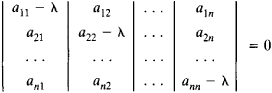The determinant on the left-hand side of the characteristic equation is obtained by subtracting λ from the diagonal elements of the matrix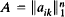. This determinant is a polynomial in λ and is called the characteristic polynomial.

The explicit form of the equation is (–λ)n + S1(–λ)n – 1 + S2(–λ)n – 2 + · · · + Sn = 0. Here, S1 = a11 + a22 + · · · + ann is the trace of the matrix; S2, is the sum of all minors of order two, that is, of all minors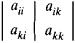where i < k; S3 through Sn – 1 are defined correspondingly; and Sn is the determinant of the matrix A.

The roots λ1, λ2, . . ., λn of the characteristic equation are called the eigenvalues of A. If A is real symmetric (more generally, Hermitian symmetric), then the λk are real. If A is real and skew symmetric, then the λk are pure imaginary. If A is orthogonal (more generally, unitary), then all | λk| = 1.

Characteristic equations are encountered in a great many areas of mathematics, physics, mechanics, and engineering. Because of their application in astronomy to the determination of secular perturbations of planets, they are also called secular equations.

(2) The characteristic equation of a linear differential equation with constant coefficients a0y(n) + a1y(n – 1) + · · · + a(n – 1)y′ + any = 0 is the algebraic equation obtained from this differential equation by replacing y and its derivatives by suitable powers of λ, that is, the equation a0λn + a1λn – 1 + · · · + an – 1 λ + an = 0. We are led to this equation if we look for solutions of the given differential equation that have the form y = ceλx. For a system of linear differential equations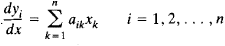the characteristic equation is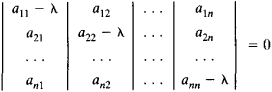and thus coincides with the characteristic equation of the matrix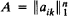whose elements are the coefficients of the equations of the system.

References in periodicals archive ?
In fact, it is well known  that stability of solutions of the linear differential equation (2.1) can be reduced to an analysis of the characteristic equation, which in this case exhibits the following form:
where [U.sub.0] is the initial capacitor voltage; [p.sub.1] and [p.sub.2] are the roots of the characteristic equation of this circuit:
analytical relations describing singular stress fields (10) in the interface plane and characteristic equation (11) can be determined .
which leads to the following characteristic equation for the eigenvalue problem ((16)-(17)):
The proposed method is based on the roots of a characteristic equation involved in finding probability distribution at a prearrival epoch.
Substituting the last equation of (87) into the boundary condition given by the second equation of (86) yields the characteristic equation
For [P.sub.01], the characteristic equation always has a positive root [r.sub.p].
To study the local stability of the disease-free equilibrium [E.sup.0], we linearized system (1) about this point and obtained the characteristic equation, given by the following determinant:
Conditions are formulated in terms of a characteristic equation associated with the system.
A mathematical characteristic equation has been defined by using the data related to the electrical arc current and voltage characteristic and curve fitting methods with the help of Matlab curve fitting toolbox.
The local stability of the equilibrium point is determined by the roots of the characteristic equation, and the equilibrium point is stable if the all the roots of the characteristic equation have negative real parts.

Site: Follow: Share:
Open / Close